MORE IN Engineering Mechanics
MU First Year Engineering (Semester 1)
Engineering Mechanics
December 2011
Total marks: --
Total time: --
INSTRUCTIONS
(1) Assume appropriate data and state your reasons
(2) Marks are given to the right of every question
(3) Draw neat diagrams wherever necessary

Solve any four of the following:-
1(a) Resolve 15 kN force acting at 'A' into two parallel components at B & C.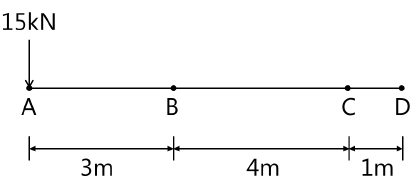5 M
1(b) Derive an expression for centrifugal tension in flat belt drive.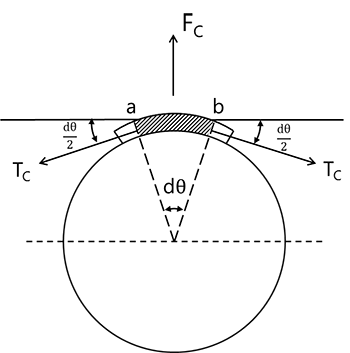5 M
1(c) Find 'P' required to accelerate the block shown in figure below at 2.5m/sec2. Take μ=0.3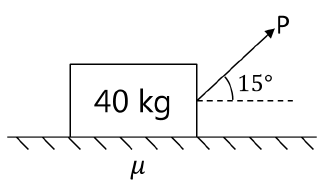5 M
1(d) A particle moves in X-Y plane and its position is given by
r=(3t)i+(4t-3t2)j
where r is the position vector of the particle measured at time 't' seconds.Find the radius of curvature of its path and normal and tangent components of acceleration when it crosses X-axis again.
5 M
1(e) Write short notes on the following:
(i) Classification of truss.
(ii) Assumptions made in the analysis of perfect truss.
5 M

2(a) Find the resultant of coplanar force system given below and locate the same on AB with consideration of applied moment of 4800 N-mm.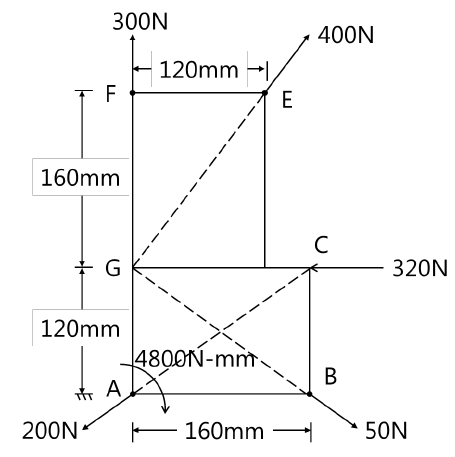10 M
2(b) If the link CD is rotating at 5 rad/sec anticlockwise, determine the angular velocity of link AB at the instant shown.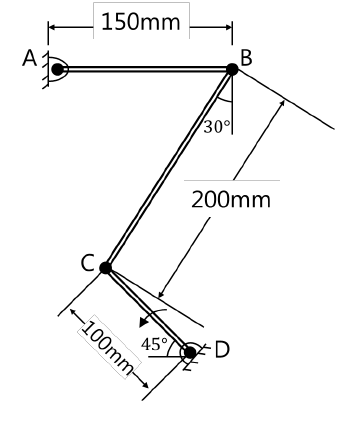10 M

3(a) Locate the centroid of the shaded area as shown in figure. Also determine area moment of inertia of shaded area about its centroidal X-axis.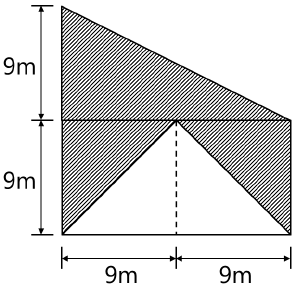10 M
3(b) Figure shows acceleration-time diagram for rectilinear motion. Construct velocity-time and displacement-time diagrams for the motion assuming that the motions starts with initial velocity of 5 m/sec from starting point.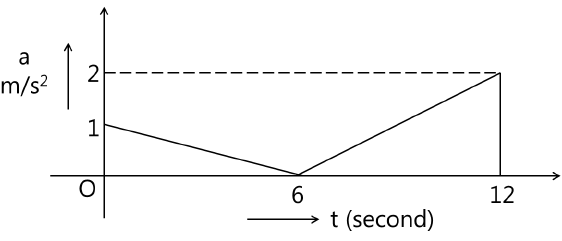10 M

4(a) What should be the value of θ so that the motion of block 'A' impend down the plane? The coefficient of friction μ for all the surfaces is 1/3.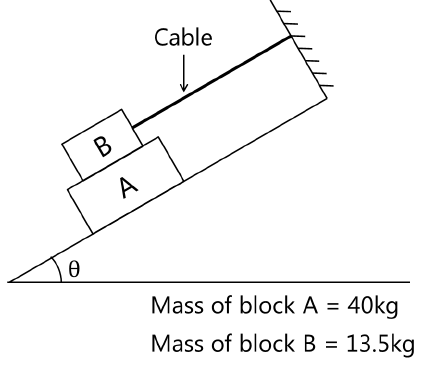10 M
4(b) Two smooth spheres A and B having a mass of 2 kg and 4 kg respectively collide with initial velocities as shown in figure. If the co-efficient of restitution for the spheres is e=0.8, determine the velocities of each sphere after collision and their directions.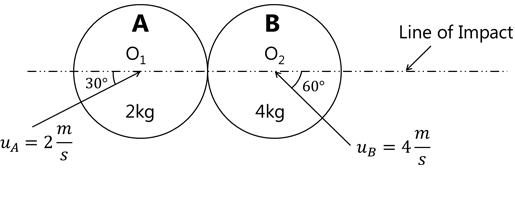10 M

5(a) Find analytically the support reaction at B and load P for the beam as shown in figure if reaction at support 'A' is zero.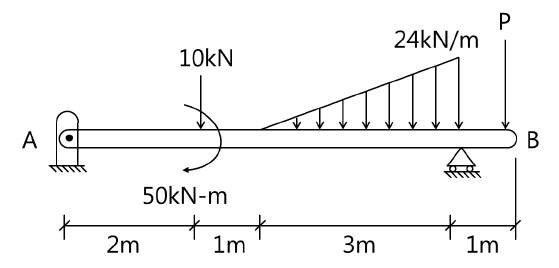10 M
5(b) Determine the weight 'W' required to bring the system in the following figure to stop in 5 second if at the instant as shown, 500 N block is moving down at 3 m/sec. The pulley is frictionless.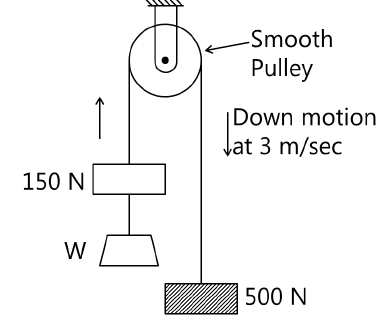10 M

6(a) A truss is loaded and supported as shown, find-
(i) Reactions at A & F (3 marks)
(ii) Forces in all members by method of joint. (9 marks)
(iii) Verify the forces in members CE, CD and BD by method of section. (3 marks)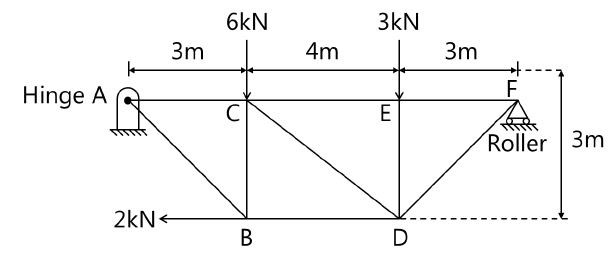15 M
6(b) Find the power transmitted by a belt running over a pulley of 600 mm diameter at 200 rpm. The coefficient of friction between the pulley and belt is 0.25 and angle of lap is 1600 and maximum tension in belt is 2.5 kN. Neglect the centrifugal tension.
5 M

7(a) Determine the reaction at point of contact 1, 2 and 3. Assume smooth surfaces.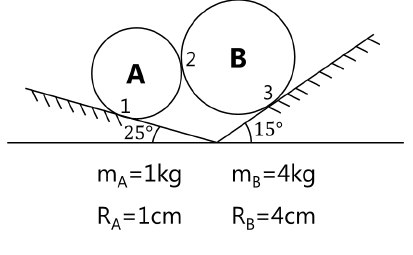10 M
7(b) Explain the following terms in short: